Algebraic Geometry Commons™

223 Full-Text Articles 268 Authors 56,862 Downloads69 Institutions

All Articles in Algebraic Geometry

223 full-text articles. Page 3 of 9.

On Vector-Valued Automorphic Forms On Bounded Symmetric Domains, 2017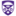The University of Western Ontario

On Vector-Valued Automorphic Forms On Bounded Symmetric Domains, Nadia Alluhaibi

Electronic Thesis and Dissertation Repository

The objective of the study is to investigate the behaviour of the inner products of vector-valued Poincare series, for large weight, associated to submanifolds of a quotient of the complex unit ball and how vector-valued automorphic forms could be constructed via Poincare series. In addition, it provides a proof of that vector-valued Poincare series on an irreducible bounded symmetric domain span the space of vector-valued automorphic forms.

2017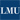University High School

Polygons, Pillars And Pavilions: Discovering Connections Between Geometry And Architecture, Sean Patrick Madden

Journal of Catholic Education

Crowning the second semester of geometry, taught within a Catholic middle school, the author's students explored connections between the geometry of regular polygons and architecture of local buildings. They went on to explore how these principles apply famous buildings around the world such as the monuments of Washington, D.C. and the elliptical piazza of Saint Peter's Basilica at Vatican City within Rome, Italy.

Classification Of Rectifying Space-Like Submanifolds In Pseudo-Euclidean Spaces, 2017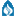Michigan State University

Classification Of Rectifying Space-Like Submanifolds In Pseudo-Euclidean Spaces, Bang Yen Chan, Yun Oh

Faculty Publications

The notions of rectifying subspaces and of rectifying submanifolds were introduced in [B.-Y. Chen, Int. Electron. J. Geom 9 (2016), no. 2, 1–8]. More precisely, a submanifold in a Euclidean m-space Em is called a rectifying submanifold if its position vector field always lies in its rectifying subspace. Several fundamental properties and classification of rectifying submanifolds in Euclidean space were obtained in [B.-Y. Chen, op. cit.]. In this present article, we extend the results in [B.-Y. Chen, op. cit.] to rectifying space- like submanifolds in a pseudo-Euclidean space with arbitrary codimension. In particular, we completely classify ...

Session A-3: Three-Act Math Tasks, 2017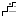Illinois Mathematics and Science Academy

Session A-3: Three-Act Math Tasks, Lindsey Herlehy

Professional Learning Day

Participants will engage in a Three-Act Math task highlighting the application of properties of geometrical figures. Developed by Dan Meyer, an innovative and highly regarded mathematics instructor, Three-Act Math tasks utilize pedagogical skills that elicit student curiosity, collaboration and questioning. By posing a mathematical problem through active storytelling, this instructional approach redefines real-world mathematics and clarifies the role that a student plays in the learning process. Participants will be given multiple resources where they can access Three-Act Math tasks appropriate for upper elementary grades through Algebra and Geometry courses.

Classification Of Book Representations Of K6, 2017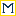Merrimack College

Classification Of Book Representations Of K6, Dana Rowland

Mathematics Faculty Publications

A book representation of a graph is a particular way of embedding a graph in three dimensional space so that the vertices lie on a circle and the edges are chords on disjoint topological disks. We describe a set of operations on book representations that preserves ambient isotopy, and apply these operations to K6, the complete graph with six vertices. We prove there are exactly 59 distinct book representations for K6, and we identify the number and type of knotted and linked cycles in each representation. We show that book representations of K6 contain between one and seven links, and ...

Drawing A Triangle On The Thurston Model Of Hyperbolic Space, 2017Loyola Marymount University

Drawing A Triangle On The Thurston Model Of Hyperbolic Space, Curtis D. Bennett, Blake Mellor, Patrick D. Shanahan

Blake Mellor

In looking at a common physical model of the hyperbolic plane, the authors encountered surprising difficulties in drawing a large triangle. Understanding these difficulties leads to an intriguing exploration of the geometry of the Thurston model of the hyperbolic plane. In this exploration we encounter topics ranging from combinatorics and Pick’s Theorem to differential geometry and the Gauss-Bonnet Theorem.

2017Loyola Marymount University

Linked Exact Triples Of Triangulated Categories And A Calculus Of T-Structures, Michael Berg

Michael Berg

We introduce a new formalism of exact triples of triangulated categories arranged in certain types of diagrams. We prove that these arrangements are well-behaved relative to the process of gluing and ungluing t-structures defined on the indicated categories and we connect our con. structs to· a problem (from number theory) involving derived categories. We also briefly address a possible connection with a result of R. Thomason.

The Jones Polynomial Of An Almost Alternating Link, 2017Vassar College

The Jones Polynomial Of An Almost Alternating Link, Adam M. Lowrance, Dean Spyropoulos

Faculty Research and Reports

No abstract provided.

Random Tropical Curves, 2017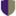Harvey Mudd College

Random Tropical Curves, Magda L. Hlavacek

HMC Senior Theses

In the setting of tropical mathematics, geometric objects are rich with inherent combinatorial structure. For example, each polynomial $p(x,y)$ in the tropical setting corresponds to a tropical curve; these tropical curves correspond to unbounded graphs embedded in $\R^2$. Each of these graphs is dual to a particular subdivision of its Newton polytope; we classify tropical curves by combinatorial type based on these corresponding subdivisions. In this thesis, we aim to gain an understanding of the likeliness of the combinatorial type of a randomly chosen tropical curve by using methods from polytope geometry. We focus on tropical curves ...

2017Harvey Mudd College

Tropical Derivation Of Cohomology Ring Of Heavy/Light Hassett Spaces, Shiyue Li

HMC Senior Theses

The cohomology of moduli spaces of curves has been extensively studied in classical algebraic geometry. The emergent field of tropical geometry gives new views and combinatorial tools for treating these classical problems. In particular, we study the cohomology of heavy/light Hassett spaces, moduli spaces of heavy/light weighted stable curves, denoted as $\calm_{g, w}$ for a particular genus $g$ and a weight vector $w \in (0, 1]^n$ using tropical geometry. We survey and build on the work of \citet{Cavalieri2014}, which proved that tropical compactification is a \textit{wonderful} compactification of the complement of hyperplane arrangement for ...

A Categorical Formulation Of Algebraic Geometry, 2017University of Massachusetts Amherst

A Categorical Formulation Of Algebraic Geometry, Bradley Willocks

Doctoral Dissertations

We construct a category, $\Omega$, of which the objects are pointed categories and the arrows are pointed correspondences. The notion of a spec datum" is introduced, as a certain relation between categories, of which one has been given a Grothendieck topology. A geometry" is interpreted as a sub-category of $\Omega$, and a formalism is given by which such a subcategory is to be associated to a spec datum, reflecting the standard construction of the category of schemes from the category of rings by affine charts.

Chow's Theorem, 2017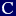Colby College

Chow's Theorem, Yohannes D. Asega

Honors Theses

We present the proof of Chow's theorem as a corollary to J.P.-Serre's GAGA correspondence theorem after introducing the necessary prerequisites. Finally, we discuss consequences of Chow's theorem.

A Journey To Fuzzy Rings, 2017Georgia Southern University

A Journey To Fuzzy Rings, Brett T. Ernst

Electronic Theses and Dissertations

Enumerative geometry is a very old branch of algebraic geometry. In this thesis, we will describe several classical problems in enumerative geometry and their solutions in order to motivate the introduction of tropical geometry. Finally, fuzzy rings, a powerful algebraic framework for tropical and algebraic geometry is introduced.

Characterization Of Rectifying And Sphere Curves In R^3, 2017Andrews University

Characterization Of Rectifying And Sphere Curves In R^3, Yun Oh, Julie Logan

Faculty Publications

Studies of curves in 3D-space have been developed by many geometers and it is known that any regular curve in 3D space is completely determined by its curvature and torsion, up to position. Many results have been found to characterize various types of space curves in terms of conditions on the ratio of torsion to curvature. Under an extracondition on the constant curvature, Y.L. Seo and Y. M. Oh found the series solution when the ratio of torsion to curvature is a linear function. Furthermore, this solution is known to be a rectifying curve by B. Y. Chen’s ...

2016The University of Western Ontario

Computation Of Real Radical Ideals By Semidefinite Programming And Iterative Methods, Fei Wang

Electronic Thesis and Dissertation Repository

Systems of polynomial equations with approximate real coefficients arise frequently as models in applications in science and engineering. In the case of a system with finitely many real solutions (the $0$ dimensional case), an equivalent system generates the so-called real radical ideal of the system. In this case the equivalent real radical system has only real (i.e., no non-real) roots and no multiple roots. Such systems have obvious advantages in applications, including not having to deal with a potentially large number of non-physical complex roots, or with the ill-conditioning associated with roots with multiplicity. There is a corresponding, but ...

2016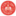University of Dayton

On The Perfect Reconstruction Of The Structure Of Dynamic Networks, Alan Veliz-Cuba

Annual Symposium on Biomathematics and Ecology: Education and Research

No abstract provided.

Non-Commutative Automorphisms Of Bounded Non-Commutative Domains, 2016Washington University in St Louis

Non-Commutative Automorphisms Of Bounded Non-Commutative Domains, John E. Mccarthy, Richard M. Timoney

Mathematics Faculty Publications

We establish rigidity (or uniqueness) theorems for non-commutative (NC) automorphisms that are natural extensions of classical results of H. Cartan and are improvements of recent results. We apply our results to NC domains consisting of unit balls of rectangular matrices.

2016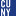The Graduate Center, City University of New York

On The Free And G-Saturated Weight Monoids Of Smooth Affine Spherical Varieties For G=Sl(N), Won Geun Kim

All Dissertations, Theses, and Capstone Projects

Let $X$ be an affine algebraic variety over $\mathbb{C}$ equipped with an action of a connected reductive group $G$. The weight monoid $\Gamma(X)$ of $X$ is the set of isomorphism classes of irreducible representations of $G$ that occur in the coordinate ring $\mathbb{C}[X]$ of $X$. Losev has shown that if $X$ is a smooth affine spherical variety, that is, if $X$ is smooth and $\mathbb{C}[X]$ is multiplicity-free as a representation of $G$, then $\Gamma(X)$ determines $X$ up to equivariant automorphism.

Pezzini and Van Steirteghem have recently obtained a combinatorial characterization of the weight ...

Critical Groups Of Graphs With Dihedral Actions Ii, 2016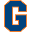Gettysburg College

Critical Groups Of Graphs With Dihedral Actions Ii, Darren B. Glass

Math Faculty Publications

In this paper we consider the critical group of finite connected graphs which admit harmonic actions by the dihedral group Dn, extending earlier work by the author and Criel Merino. In particular, we show that the critical group of such a graph can be decomposed in terms of the critical groups of the quotients of the graph by certain subgroups of the automorphism group. This is analogous to a theorem of Kani and Rosen which decomposes the Jacobians of algebraic curves with a Dn-action.

2016The University of Western Ontario

K-Theory Of Root Stacks And Its Application To Equivariant K-Theory, Ivan Kobyzev

Electronic Thesis and Dissertation Repository

We give a definition of a root stack and describe its most basic properties. Then we recall the necessary background (Abhyankar’s lemma, Chevalley-Shephard-Todd theorem, Luna’s etale slice theorem) and prove that under some conditions a quotient stack is a root stack. Then we compute G-theory and K-theory of a root stack. These results are used to formulate the theorem on equivariant algebraic K-theory of schemes.